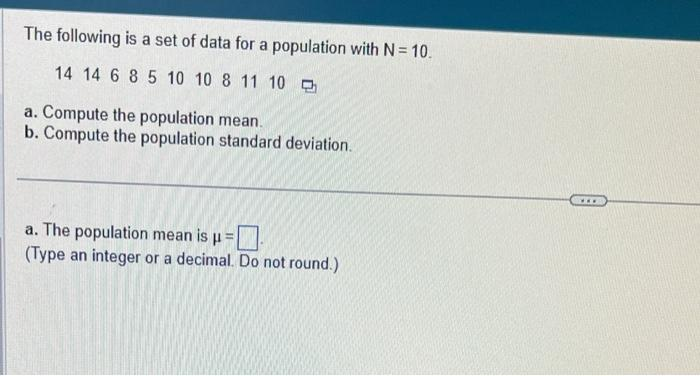Home / Expert Answers / Statistics and Probability / the-following-is-a-set-of-data-for-a-population-with-mathrm-n-10-14-begin-array-lllll-pa134

# (Solved): The following is a set of data for a population with $$\mathrm{N}=10$$. $$14 \begin{array}{lllll ...The following is a set of data for a population with \( \mathrm{N}=10$$. $$14 \begin{array}{lllllllll}14 & 6 & 8 & 5 & 10 & 10 & 8 & 11 & 10\end{array}$$ a. Compute the population mean. b. Compute the population standard deviation. a. The population mean is $$\mu=$$ (Type an integer or a decimal. Do not round.)

We have an Answer from Expert